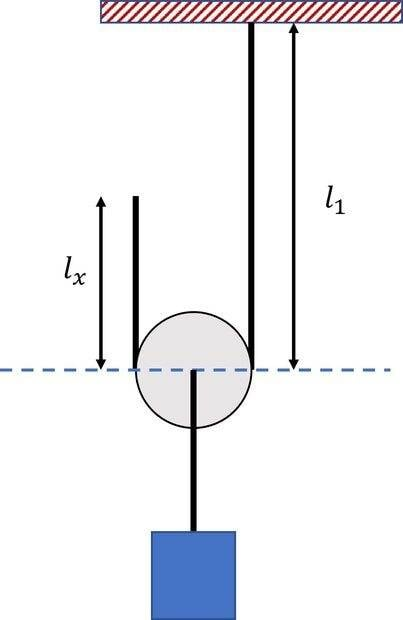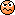# Displacement of Hanging Mass - Simple Pulley System

• B
• erobz
In summary, the conversation revolved around a physical problem where the length of a cord and the position of a hanging mass were related by a constraint. The speaker struggled to understand why a change in the length of one part of the cord resulted in a change in the position of the mass. Another person explained that this was due to the lack of a fixed coordinate system, and once a coordinate system was established, the problem became clearer.

#### erobz

Homework Helper
Gold Member
I'm having some kind of mental block.If I extend ##l_x## by ##\delta## ,I expect the hanging mass to move ##\frac{ \delta}{2}##.

I can't figure out how this is the case from the constraint:

$$l_x+l_1=C$$

##C## is an arbitrary length

I keep getting that ##l_1## changes by ##\delta##, but that must mean the height of the mass changes by ##\delta##...Last edited:
I suspect you need to differentiate between the distance you pull the left hand cord up, ##\delta##, and the increase in distance from that point to the pulley, ##\frac \delta 2##.

•topsquark
Call the distance of the left end of the cord from the ceiling ##y##. Then ##l_x=l_1-y## and ##l_x=C-l_1## from the constraint given by the fixed length of the entire cord. Then you can eliminate the somewhat inconvenient quantity ##l_1## in favor of ##y## which has a well defined meaning of a coordinate, i.e., it's a length measured from a fixed reference point (the ceiling):
$$C-l_1=l_1-y \; \Rightarrow \; L_1=\frac{1}{2}(C+y).$$
So if you change ##y## by ##\delta## (which is the same as changing ##l_x## by ##-\delta##) ##L_1## (the distance of the pulley from the ceiling) changes by ##\delta/2##.

•topsquark and erobz
vanhees71 said:
Then you can eliminate the somewhat inconvenient quantity ##l_1## in favor of ##y## which has a well defined meaning of a coordinate, i.e., it's a length measured from a fixed reference point (the ceiling):
The addition of the coordinate ##y## and the weirdness seems to vanish right in front of my eyes. Amazing!

Last edited:
•vanhees71
My key failure was that I had not defined a fixed coordinate system. I basically was trying to measure distances relative the datum at the center of the pulley...a transformation not so obvious to me.

•vanhees71

## 1. What is a displacement of hanging mass in a simple pulley system?

The displacement of a hanging mass in a simple pulley system refers to the change in position of the mass as it is lifted or lowered by the pulley. This displacement is typically measured in meters or centimeters.

## 2. How does a simple pulley system work?

A simple pulley system consists of a rope or cable wrapped around a wheel or pulley, with one end attached to a fixed point and the other end attached to a load. When one end of the rope is pulled, the load is lifted or lowered depending on the direction of the force applied.

## 3. What factors affect the displacement of a hanging mass in a simple pulley system?

The displacement of a hanging mass in a simple pulley system is affected by the mass of the load, the length of the rope, the angle of the rope, and the resistance or friction in the pulley system.

## 4. How is the displacement of a hanging mass in a simple pulley system calculated?

The displacement of a hanging mass in a simple pulley system can be calculated using the formula d = F * d, where d is the displacement, F is the force applied, and d is the length of the rope.

## 5. What are some real-world applications of the displacement of hanging mass in a simple pulley system?

The displacement of hanging mass in a simple pulley system is commonly used in lifting heavy objects, such as in construction, and in mechanical systems such as elevators and cranes. It is also used in physics experiments to study the relationship between force, displacement, and work.